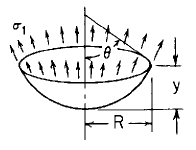Related Resources: pressure-vessel

### Spherical Cylinder Stress and Deflection Own weight Formula and Calculator

Spherical Cylinder Stress and Deflection Own weight, δ force/unit volume; tangential top edge support Equation and Calculator.

Per. Roarks Formulas for Stress and Strain for membrane stresses and deformations in thin-walled pressure vessels.

 Spherical Cylinder Stress and DeflectionOwn weight, δ force/unit volume; tangential top edge supportDeflection and Stress Own weight, δ force/unit volume; tangential top edge support.

For R2 / t > 10

Meridional StressCircumferential Hoop StressMaximum tensile stressat θ = 0°

 σ2 = 0 at θ = 51.83°Change in height dimension yRotation of a meridian from its unloaded positionWhere used:
E = Modulus of Elasticity (lbs/in2)
v = Poisson's ratio
δ = Density (lbs/in3)
σ1,2 = Stress, (lbs/in2)
R = Distance as indicated (in)
y = Depth as indicated (in), Must be equal too or less than d
d = Depth of liquid (in)
t = Wall thickness (in)
θ = Angle (deg.)
ψ = Rotation of a meridian from its unloaded position, positive when that meridional rotation represents an increase in ΔR when y or θ increases;

(Note: There is a discontinuity in the rate of increase in fluid pressure at the top of the liquid. This leads to some bending in this region and is indicated by a discrepancy in the two expressions for the meridional slope at y = d.)

Reference:

Roarks Formulas for Stress and Strain, 7th Edition